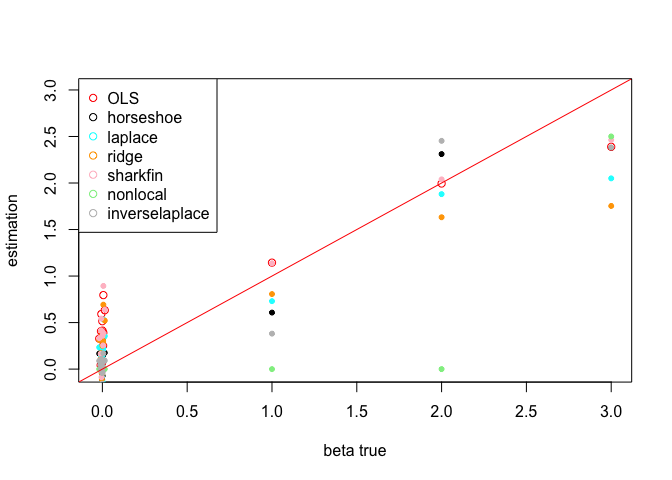# Demo of the bayeslm package

In this vignette, we show how to use bayeslm to sample coefficients for a Gaussian linear regression with a number of different prior distributions.

library(bayeslm)

# Generate data

set.seed(200)
p = 20
n = 100
kappa = 1.25
beta_true = c(c(1,2,3),rnorm(p-3,0,0.01))
sig_true = kappa*sqrt(sum(beta_true^2))
x = matrix(rnorm(p*n),n,p)
y = x %*% beta_true + sig_true * rnorm(n)
x = as.matrix(x)
y = as.matrix(y)
data = data.frame(x = x, y = y)

## Modeling $$Y \mid X = x$$ using linear regression

### OLS

First, we run OLS and inspect the estimated coefficients

fitOLS = lm(y~x-1)
coef(fitOLS)
#>          x1          x2          x3          x4          x5          x6
#>  1.14415549  1.99442217  2.38953931 -0.73055959 -0.71840494  0.25239729
#>          x7          x8          x9         x10         x11         x12
#>  0.39044226  0.41366834 -0.39830816  0.04170669 -0.20976664 -0.24878570
#>         x13         x14         x15         x16         x17         x18
#>  0.63260575  0.07410838  0.40714461 -0.18807163  0.79535530  0.32748131
#>         x19         x20
#>  0.59214904  0.51461938

### bayeslm

#### Updating coefficients individually

The bayeslm sampler can group coefficients into blocks and sample several coefficients at once. Below, we simply place every coefficient into its own block.

block_vec = rep(1, p)

Now, we run bayeslm on six different priors and store their estimated coefficients

# Horseshoe prior
fit1 = bayeslm(y, x, prior = 'horseshoe', icept = FALSE,
block_vec = block_vec, N = 10000, burnin=2000)
beta_est1 = colMeans(fit1$beta) # Laplace prior fit2 = bayeslm(y, x, prior = 'laplace', icept = FALSE, block_vec = block_vec, N = 10000, burnin=2000) beta_est2 = colMeans(fit2$beta)

# Ridge prior
fit3 = bayeslm(y, x, prior = 'ridge', icept = FALSE,
block_vec = block_vec, N = 10000, burnin=2000)
beta_est3 = colMeans(fit3$beta) # "Sharkfin" prior fit4 = bayeslm(y, x, prior = 'sharkfin', icept = FALSE, block_vec = block_vec, N = 10000, burnin=2000) beta_est4 = colMeans(fit4$beta)

# "Non-local" prior
fit5 = bayeslm(y, x, prior = 'nonlocal', icept = FALSE,
block_vec = block_vec, N = 10000, burnin=2000)
beta_est5 = colMeans(fit5$beta) # Inverse laplace prior fit6 = bayeslm(y, x, prior = 'inverselaplace', lambda = 0.01, icept = FALSE, block_vec = block_vec, N = 10000, burnin=2000) beta_est6 = colMeans(fit6$beta)

And we plot the posterior distribution of the regression coefficients, along with the OLS estimates, against the true simulated coefficients.

plot(NULL,xlim=range(beta_true),ylim=range(beta_true),
xlab = "beta true", ylab = "estimation", )
points(beta_true,beta_est1,pch=20)
points(beta_true,fitOLS$coef,col='red') points(beta_true,beta_est2,pch=20,col='cyan') points(beta_true,beta_est3,pch=20,col='orange') points(beta_true,beta_est4,pch=20,col='pink') points(beta_true,beta_est5,pch=20,col='lightgreen') points(beta_true,beta_est6,pch=20,col='grey') legend("topleft", c("OLS", "horseshoe", "laplace", "ridge", "sharkfin", "nonlocal", "inverselaplace"), col = c("red", "black", "cyan", "orange", "pink", "lightgreen", "grey"), pch = rep(1, 7)) abline(0,1,col='red')We can also compare the root mean squared error (RMSE) for each prior rmseOLS = sqrt(sum((fitOLS$coef-beta_true)^2))
rmse1 = sqrt(sum((beta_est1-beta_true)^2))
rmse2 = sqrt(sum((beta_est2-beta_true)^2))
rmse3 = sqrt(sum((beta_est3-beta_true)^2))
rmse4 = sqrt(sum((beta_est4-beta_true)^2))
rmse5 = sqrt(sum((beta_est5-beta_true)^2))
rmse6 = sqrt(sum((beta_est6-beta_true)^2))
print(cbind(ols = rmseOLS, hs = rmse1,laplace = rmse2, ridge = rmse3,
sharkfin = rmse4,nonlocal = rmse5, inverselaplace = rmse6))
#>           ols       hs  laplace    ridge sharkfin nonlocal inverselaplace
#> [1,] 2.013695 1.082114 1.463458 1.954048 1.990372 2.291407       1.058102

#### Updating coefficients in blocks

Here, we demonstrate:

1. How to place several coefficients in the same block for the slice-within-Gibbs sampler
2. How to use formula notation (y ~ x) in the bayeslm library
# Put the first two coefficients in one elliptical sampling block
block_vec2 = c(2, rep(1, p-2))
fitb = bayeslm(y ~ x - 1, data = data, prior = 'horseshoe',
block_vec = block_vec2, N = 10000, burnin = 2000)
#> horseshoe prior
#> fixed running time 0.000402834
#> sampling time 0.223155
summary(fitb)
#> Average number of rejections before one acceptance :
#> 31.2156
#> Summary of beta draws
#>    based on 8000 valid draws (number of burn in is 2000)
#> Summary of Posterior draws
#>  Moments
#>        mean std dev eff sample size
#> x1   0.6403    0.52             764
#> x2   2.1377    0.68             618 ***
#> x3   2.6466    0.47            2362 ***
#> x4  -0.2519    0.39            1529
#> x5  -0.2663    0.37            1662
#> x6   0.0351    0.24            5648
#> x7   0.1552    0.33            2617
#> x8   0.1484    0.32            2675
#> x9  -0.2187    0.36            1703
#> x10  0.0558    0.25            4862
#> x11 -0.0727    0.26            4570
#> x12 -0.0597    0.26            4983
#> x13  0.2759    0.37            1184
#> x14  0.0089    0.29            7268
#> x15  0.1151    0.28            2807
#> x16 -0.0355    0.28            6747
#> x17  0.3939    0.46            1102
#> x18  0.0882    0.25            3785
#> x19  0.2470    0.37            1680
#> x20  0.1576    0.32            2567
#> Signif. codes: 0 '***' 0.001 '**' 0.01 '*' 0.05 '.' 0.1 ' ' 1
#>
#> Summary of sigma draws
#> Mean of standard deviation is  4.555075
#> S.d. of standard deviation samples is  0.3624706
#> Effective sample size of s.d. is  3357.107